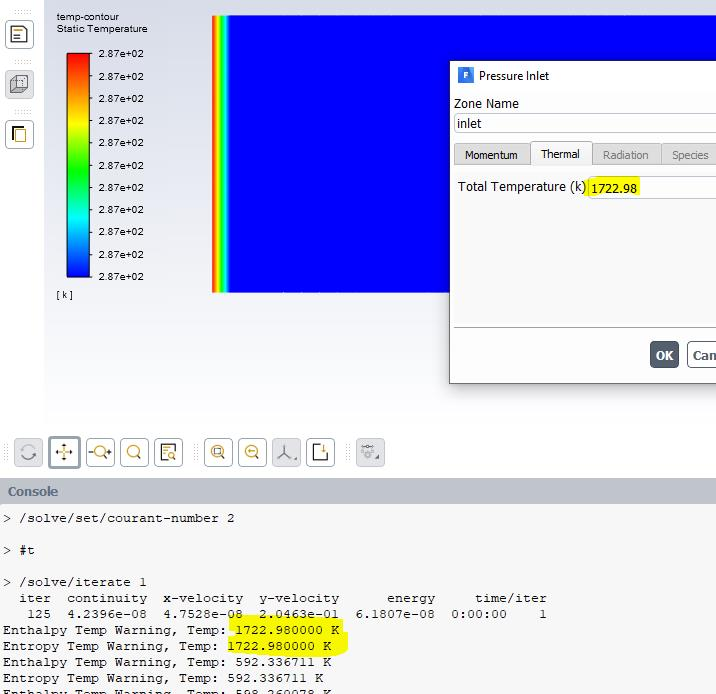CaptainConvergence
Subscriber

If instead of calculating the CP in each loop I leave it as constant:

/*

*/
/*
*/
/* ENTHALPY UDF (INTERPOLATION) */
/*
*/
/*
*/
double REAL_enthalpy(cell_t cell, Thread *thread, double Temp, double density, double P, double yi[])
{
double cp = 1004.16; // J/(Kg K)
double h = Temp * cp;

if (Temp>500){
Message("Enthalpy Temp Warning, Temp: %f Kn", Temp); // CHECK POSITION!!
}

return h; /* (J/Kg) */
}
/*
*/
/*
*/
/* ENTROPY UDF */
/*
*/
/*
*/

double REAL_entropy(cell_t cell, Thread *thread, double Temp, double density, double P, double yi[])
{
double RGAS = Runiv/MW0;
double cp = 1004.16; // J/(Kg K)
double s = cp * log(fabs(Temp / TDatum)) + RGAS * log(fabs(PDatum / P));

if (Temp>500){
Message("Entropy Temp Warning, Temp: %f Kn", Temp);
}

return s; /* (J/Kg/K) */
}

I am still having the same temperature warnings but now the static temperature does not change because the Cp remains constant (See the attached image). But there is an enthalpy calculation error because it is being calculated (apparently) with the Total Temperature.